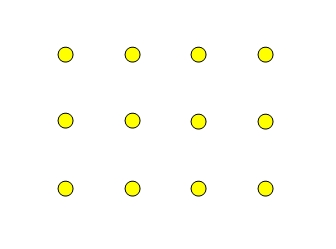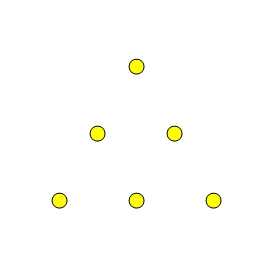Home > Patterns > Activities > Rectangular arrays

# Rectangular arrays

A rectangular array consists of a set of objects arranged in a similar way to a rectangular grid. It is one way of packing objects (e.g. eggs) efficiently.

This is an array of dots.A 3 $$\times$$ 4 array.

Activities with arrays can help students understand the rectangular grid pattern. Here are some suggestions.

• Supply students with drawings of various rectangular arrays of black dots and coloured counters.
Ask them to create their own colour patterns by placing the counters on the dots.
• Ask students to draw arrays of various sizes from memory.
• Supply students with drawings of various rectangular arrays of dots and ask them to count the number of dots in each array.
Then ask them to extend each array by one row and one column, and count the number of dots again.

Through such exercises, students will learn that the dots are aligned and equally spaced.

More advanced learners can be challenged to copy and extend a triangular grid pattern of dots.A triangular array.